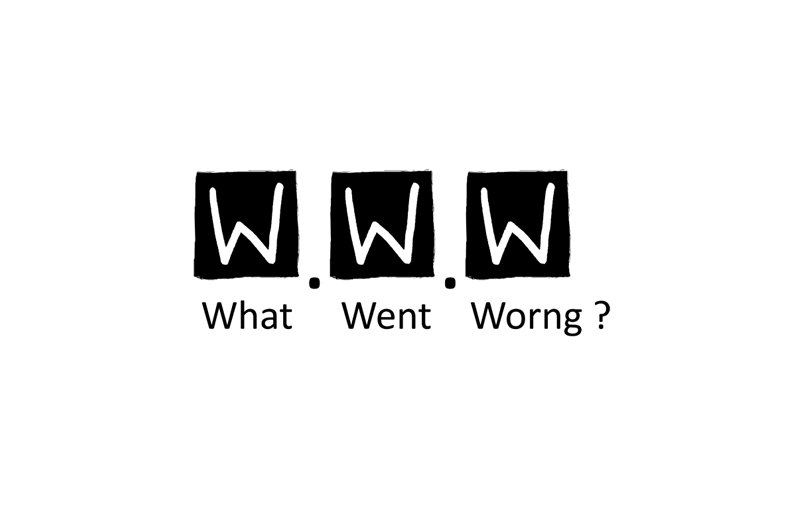# {}

### Parenthesis Brackets in JS

###### Pretty simple, isn't it?

by Jingwei Liu (@th507), Meituan.com

## Questions

`[] + [] = ?[] + {} = ?`
```[] + [] = "" [] + {} = "[object Object]"```

## Why?

• +
• valueOf
```[] + {} = [].valueOf() + {}.valueOf()```

### Primitive value

`Number, String, Boolean, undefined, null`

typecast operands before evaluation

``````
// typecast obj to boolean
!!obj
``````

### Array's primitive value

``````
var arr = [];
// still not a primitive value
arr.valueOf() === arr;
``````
``````
[] + {}
// trying to get primitive value by valueOf
= ([]).valueOf() + ({}).valueOf()
// previous attempt fails, trying typecast instead
= ([]).toString() + ({}).toString()
= "" + "[object Object]"
= "[object Object]"
``````

## Ponder this

``````
[] + {valueOf: function valueOf() { return 2}}
``````

## So far so good

``````
[] + {valueOf: function valueOf() { return 2}}
= "" + {valueOf: function valueOf() { return 2}}.valueOf()
= "" + 2
= "2"
``````

## Ponder this

``````
{valueOf: function valueOf() { return 2}} + {valueOf: function valueOf() { return 2}}
``````

## What?

``````
{valueOf: function valueOf() { return 2}} + {valueOf: function valueOf() { return 2}}

= 2
``````http://watchdogwire.com/florida/2013/08/26/book-review-what-went-wrong-by-jerome-corsi/

## Code block

`{ StatementList }` Return the result of evaluating StatementList.

``````
var a = 1, b = 2;
{a = 2; b = 3};
console.log(a, b);
``````

## Code block is everywhere

``````
// this is OK
[].toString();
// this will throw an error
{}.hasOwnProperty();

// this is OK. Wait, what?
{}hasOwnProperty();
``````

## Code block is everywhere

The leading {} is treated as a code block in browsers.

``````
{}.hasOwnProperty();
// equals to
.hasOwnProperty();
``````

``````
{}hasOwnProperty();
// equals to
hasOwnProperty();

// Chrome has an window.hasOwnProperty :)
``````

## Be careful withcode block

``````
// There are many ways to fool the browser
// so that {} won't be treated as a block
// wrap it in ()
({}).hasOwnProperty();
// add something in front of {}
"",{}.hasProperty();
``````

Compression tools "fix" this for us.

## Labelled Statement

A statement may be prefixed by a label.

Labelled statements are only used in conjunction with labelled break and continue statements.

``````
loop: for (var i = 0; i < 1000; i++) {
if (i === 233) break loop;
}
// i = 233
console.log(i);
``````

## Labelled Statement

Almost any statement can be prefixed by a label.

``````
pointless: var a = 1;
``````

Label doesn't do anything in this case.

## So we have...

``````
{} + []
// processing code block
= +[]
// trying to get primitive value
= + [].valueOf()
= + [].toString()
= + ""
// typecast
= Number("")
= 0
``````

## Revealing the trick

``````
{valueOf: function valueOf() { return 2}} + {valueOf: function valueOf() { return 2}}
``````

The first part is treated as a code block with a label and a statement inside.

``````
// This first part
{valueOf: function valueOf() { return 2}}
// { label: statement }
// label: "valueOf"
// statement: function valueOf() { return 2}
``````

It doesn't return anything.

## Revealing the trick

``````
{valueOf: function valueOf() { return 2}} + {valueOf: function valueOf() { return 2}}
``````

The second part is an object with a customized `valueOf` method.

The answer is +2 = 2

## Similarly...

``````
{} + [] = +[] = 0 // in browser, not Node.js
{} + {} = +{} = NaN
{} + new Date = 1393349722245
[] + new Date = "Wed Feb 26 2014 01:35:28 GMT+0800 (KRAT)"
[] + + new Date = 1393349722245
``````